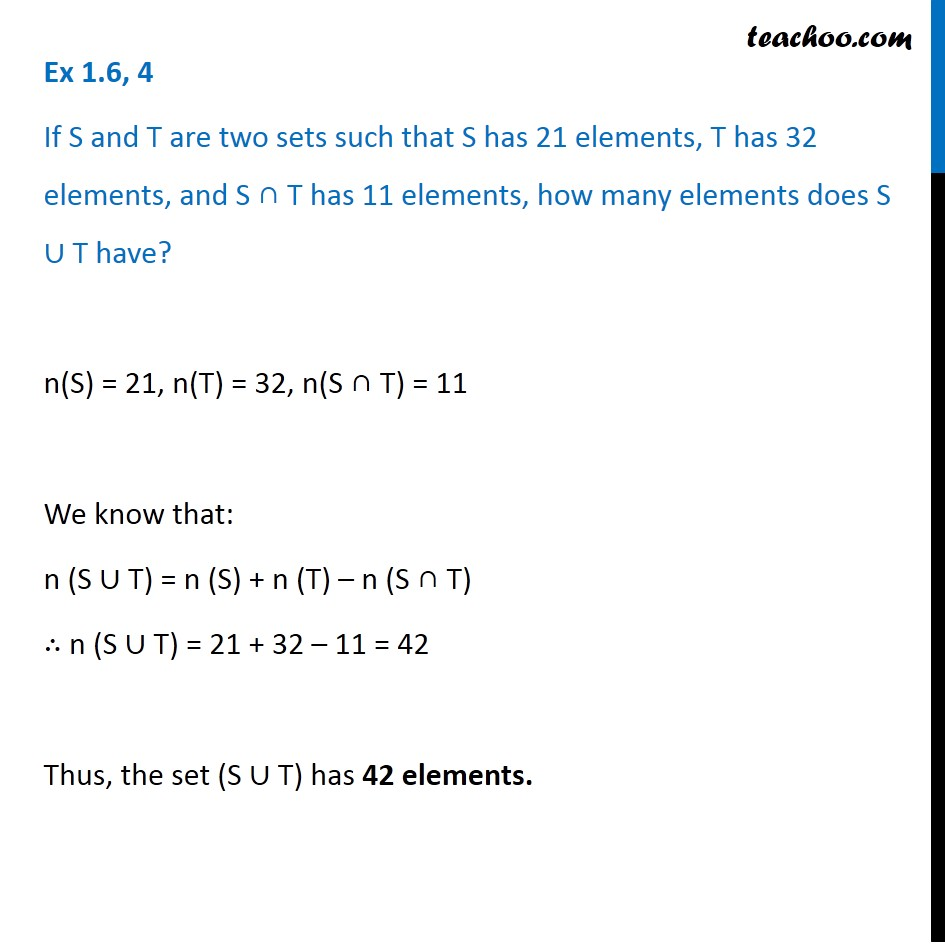1. Chapter 1 Class 11 Sets
2. Concept wise
3. Number of elements in set - 2 sets (Direct)

Transcript

Ex 1.6, 4 If S and T are two sets such that S has 21 elements, T has 32 elements, and S ∩ T has 11 elements, how many elements does S ∪ T have? n(S) = 21, n(T) = 32, n(S ∩ T) = 11 We know that: n (S ∪ T) = n (S) + n (T) – n (S ∩ T) ∴ n (S ∪ T) = 21 + 32 – 11 = 42 Thus, the set (S ∪ T) has 42 elements.

Number of elements in set - 2 sets (Direct)